# DecimalsPage 1

#### WATCH ALL SLIDES

Slide 1Place Value with Decimals

Chapter 1.2

Slide 2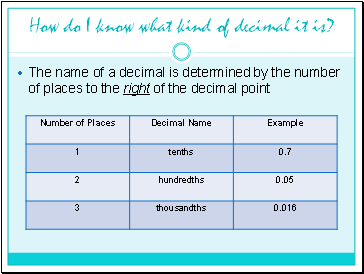## How do I know what kind of decimal it is?

The name of a decimal is determined by the number of places to the right of the decimal point

Slide 3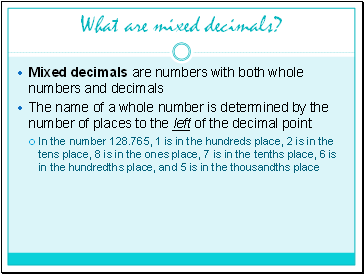## What are mixed decimals?

Mixed decimals are numbers with both whole numbers and decimals

The name of a whole number is determined by the number of places to the left of the decimal point

In the number 128.765, 1 is in the hundreds place, 2 is in the tens place, 8 is in the ones place, 7 is in the tenths place, 6 is in the hundredths place, and 5 is in the thousandths place

Slide 4## How do you read decimals?

To read a decimal correctly, first find the decimal point

Whole numbers are to the left of the decimal point; any numbers to the right of a decimal point form a decimal fraction

Say “and” for the decimal point

The decimal 2164.511 is read as “two thousand, one hundred sixty-four and five hundred eleven thousandths”

Slide 5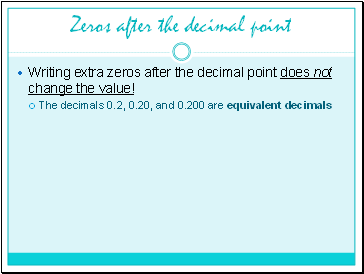## Zeros after the decimal point

Writing extra zeros after the decimal point does not change the value!

The decimals 0.2, 0.20, and 0.200 are equivalent decimals

Slide 6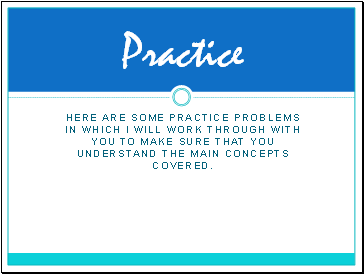## Practice

Here are some practice problems in which I will work through with you to make sure that you understand the main concepts covered.

Slide 7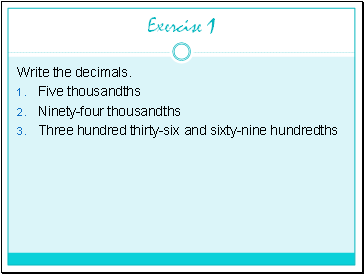Exercise 1

Write the decimals.

Five thousandths

Ninety-four thousandths

Three hundred thirty-six and sixty-nine hundredths

Slide 8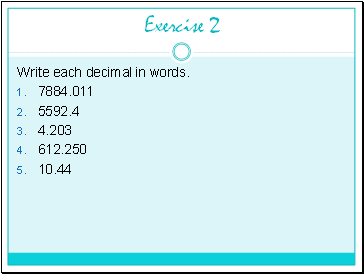Exercise 2

Write each decimal in words.

7884.011

5592.4

4.203

612.250

10.44

Slide 9Exercise 3

In what place (on the place value chart) is the underlined digit? Write the answer.

1.475

3.763

7780.215

412.407

902.103

Slide 10Exercise 4

Write a decimal that has the same number.

0.2

5.51

410.6

753.809